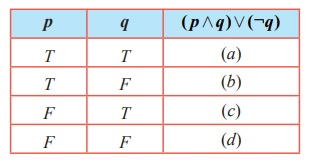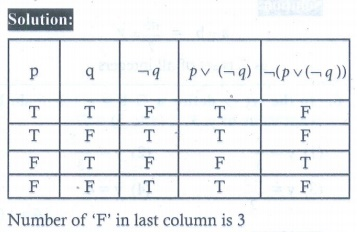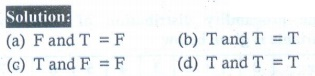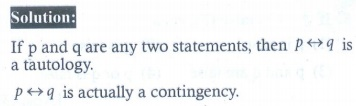Home | | Maths 12th Std | Exercise 12.3: Choose the correct answer

# Exercise 12.3: Choose the correct answer

Choose the correct or the most suitable answer from the given four alternatives. - Maths Book back answers and solution for Exercise questions - Mathematics : Discrete Mathematics

EXERCISE 12.3

Choose the correct or the most suitable answer from the given four alternatives.

1. A binary operation on a set S is a function from

(1) S  S

(2) (S×S)→ S

(3) S →(S×S)

(4)(S×S)→(S×S)

2. Subtraction is not a binary operation in

(1)

(2)

(3)

(4)3. Which one of the following is a binary operation on ?

(1) Subtraction

(2) Multiplication

(3) Division

(4) All the above

4. In the set  of real numbers ‘ * ’ is defined as follows. Which one of the following is not a binary operation on ℝ ?

(1)  b = min (a×b)

(2)  b = max (a, b)

(3)  b = a

(4)  b = ab5. The operation * defined by a  b = ab/7 is not a binary operation on

(1) +

(2)

(3)

(4) C6. In the set  defineb = a +b +ab . For what value of y, 3( y5)= 7 ?

(1) y = 2/3

(2) y = −2/3

(3) y = −3/2

(4) y = 47. If a  b = √(a2 +b2) on the real numbers then * is

(1) commutative but not associative

(2) associative but not commutative

(3) both commutative and associative

(4) neither commutative nor associative8. Which one of the following statements has the truth valueT ?

(1) sin x is an even function.

(2) Every square matrix is non-singular

(3) The product of complex number and its conjugate is purely imaginary

(4) √5 is an irrational number

9. Which one of the following statements has truth value F ?

(1) Chennai is in India or √2 is an integer

(2) Chennai is in India or √2 is an irrational number

(3) Chennai is in China or √2 is an integer

(4) Chennai is in China or √2 is an irrational number

10. If a compound statement involves 3 simple statements, then the number of rows in the truth table is

(1) 9

(2) 8

(3) 6

(4) 311. Which one is the inverse of the statement ( p  q )  ( p q) ?

(1) ( p  q )  ( p q)

(2) ¬( p q ) → ( p q)

(3) (¬ p ¬ q )  (¬ p ¬q)

(4) (¬ p ¬ q )  (¬ p ¬q)12. Which one is the contrapositive of the statement ( p  q )  r ?

(1) ¬ r → (¬ p ¬q)

(2) ¬ r → ( p q)

(3)  ( p q)

(4)  ( q r)13. The truth table for ( p  q ) ¬q is given belowWhich one of the following is true?14. In the last column of the truth table for ¬ ( p ¬q) the number of final outcomes of the truth value ' F ' are

(1) 1

(2) 2

(3) 3

(4) 415. Which one of the following is incorrect? For any two propositions p and q , we have

(1) ¬ ( p  q) ≡ ¬p ¬q

(2) ¬ ( p  q) ≡ ¬p ¬q

(3) ¬ ( p  q) ≡ ¬p ¬q

(4) ¬ (¬p ) ≡ p

16.Which one of the following is correct for the truth value of ( p  q)→ ¬ p?

(a) (b) (c) (d)

(1) T T T T

(2) F T T T

(3) F F T T

(4) T T T F17. The dual of ¬ ( p  q)  [ p ( p ¬r)] is

(1) ¬ ( p  q)  [ p ( p ¬r)]

(2) ( p  q ) [ p  ( p ¬r)]

(3) ¬ ( p  q)  [ p ( p  r)]

(4) ¬ ( p  q)  [ p ( p ¬r)]18. The proposition p  (¬p  q) is

(1) a tautology

(3) logically equivalent to p  q

(4) logically equivalent to p  q19. Determine the truth value of each of the following statements:

(a) 4 + 2 = 5 and 6 + 3 = 9

(b) 3+ 2 = 5 and 6 + 1 = 7

(b) 4 + 5 = 9 and 1+ 2 = 4

(d) 3+ 2 = 5 and 4 + 7 =11

(a) (b) (c) (d)

(1) F T F T

(2) T F T F

(3) T T F F

(4) F F T T20. Which one of the following is not true?

(1) Negation of a negation of a statement is the statement itself.

(2) If the last column of the truth table contains only T then it is a tautology.

(3) If the last column of its truth table contains only F then it is a contradiction

(4) If p and q are any two statements then p  q is a tautology.Tags : Discrete Mathematics | Mathematics , 12th Maths : UNIT 12 : Discrete Mathematics
Study Material, Lecturing Notes, Assignment, Reference, Wiki description explanation, brief detail
12th Maths : UNIT 12 : Discrete Mathematics : Exercise 12.3: Choose the correct answer | Discrete Mathematics | Mathematics